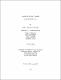2012
##### Publisher
University of Alabama Libraries
##### Abstract

Quadratic Projections is a saxophone quartet in three movements using three projected pitch collections, each paired with a separate mathematical concept for organization, pitch groupings, tonal levels, and form. The instrumentation is soprano saxophone doubling on guiro and clarinet, alto saxophone doubling on vibra-slap and bass clarinet, tenor saxophone doubling on triangle and flute, and baritone saxophone doubling on rain stick and oboe. Projection is a term used by Howard Hanson to describe the stacking of sonorities, intervals or chords, one above the other. The trichord (three-note chord) used in Quadratic Projections, consisting of one perfect fifth, one major third and one minor third, can be expressed as either a major triad or a minor triad. The mathematical concepts used are pi carried to the 20th decimal place, the Fibonacci series expressed as the golden ratio, and Pascal's triangle. The first movement pairs a minor triad with a major triad projected at the tritone, using pi to the 20th place - pi = 3.14159265358979323846. Each digit of pi determines the 21-pitch grouping. The first four digits are used to determine the tonal levels of the movement. Movement two superimposes a minor triad upon itself. The form of the second movement is based on the Fibonacci series expressed as the golden ratio, also called the divine proportion. The golden ratio is the organizing factor of the form of the movement. A texture and tempo change occurs at the golden ratio and a rhythmic change occurs at the golden ratio of the golden ratio. The third movement projects minor or major triads at the interval of a major second. The mathematical concept employed is Pascal's triangle, an array of binomial coefficients beginning with "1" at its apex. As in Pascal's triangle, the movement begins with a single instrument gradually expanding to the entire quartet. The form consists of a five-part palindrome followed by a short coda.

##### Description
Electronic Thesis or Dissertation
Music